# Algebra II : Graphing Logarithmic Functions

## Example Questions

### Example Question #1 : Graphing Logarithmic Functions

Give the-intercept of the graph of the function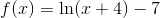to two decimal places.

Possible Answers: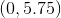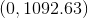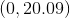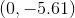The graph has no-intercept.

Correct answer:Explanation:

Setand solve: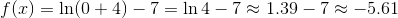The-intercept is.

### Example Question #2 : Graphing Logarithmic Functions

Give theintercept of the graph of the functionto two decimal places.

Possible Answers:

The graph has no-intercept.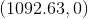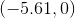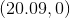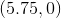Correct answer:Explanation:

Set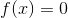and solve: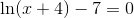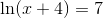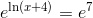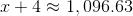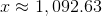The-intercept is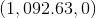.

### Example Question #3 : Graphing Logarithmic Functions

What is/are the asymptote(s) of the graph of the function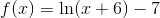?

Possible Answers: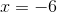and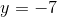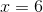andCorrect answer:Explanation:

The graph of the logarithmic function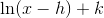has as its only asymptote the vertical line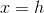Here, since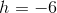, the only asymptote is the line.

### Example Question #4 : Graphing Logarithmic Functions

Which is true about the graph of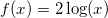?

Possible Answers:

All of the answers are correct

The domain of the function is greater than zero

When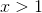,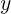is twice the size as in the equation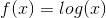None of the answers are correct

The range of the function is infinite in both directions positive and negative.

Correct answer:

All of the answers are correct

Explanation:

There is no real numberfor which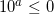Therefore in the equation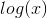,cannot be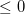However,can be infinitely large or negative.

Finally, when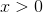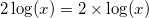or twice as large.

### Example Question #5 : Graphing Logarithmic Functions

Which of the following is true about the graph of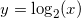Possible Answers:

It is an odd function.

It is an even function.

The range must be greater than zero.

The domain is infinite in both directions.

The graph is the mirror image of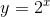flipped over the line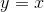Correct answer:

The graph is the mirror image offlipped over the lineExplanation:is the inverse ofand therefore the graph is simply the mirror image flipped over the line### Example Question #6 : Graphing Logarithmic Functions

Give the equation of the horizontal asymptote of the graph of the equation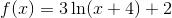.

Possible Answers: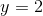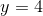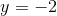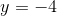The graph ofdoes not have a horizontal asymptote.

Correct answer:

The graph ofdoes not have a horizontal asymptote.

Explanation:

Let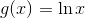In terms of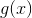,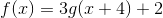This is the graph ofshifted left 4 units, stretched vertically by a factor of 3, then shifted up 2 units.

The graph ofdoes not have a horizontal asymptote; therefore, a transformation of this graph, such as that of, does not have a horizontal asymptote either.

### Example Question #7 : Graphing Logarithmic Functions

Find the equation of the vertical asymptote of the graph of the equation.

Possible Answers: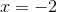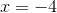Correct answer:Explanation:

Let. In terms of,.

The graph ofhas as its vertical asymptote the line of the equation. The graph ofis the result of three transformations on the graph of- a left shift of 4 units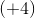, a vertical stretch (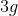), and an upward shift of 2 units (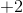). Of the three transformations, only the left shift affects the position of the vertical asymptote - the asymptote ofalso shifts left 4 units, to.

### All Algebra II Resources